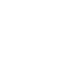# Algebra Factoring Tip

I am constantly surprised by what students tell me about what they learn in my SAT/ACT program that is not taught in their math classes.  Maybe the test writers know that and use the stuff that they know isn’t taught to create their questions!

One example is that the mean is the balancing point for a group of numbers.  The deficit created by the numbers below the mean must have the same sum as the surplus created by the numbers above the mean.  Watch the video on my home page and you’ll see a complete explanation and some examples.  Using that strategy will certainly speed you up on test day.

This past weekend I was working with a group of students (Hello, “Stars!) who were surprised by something I said.  We were talking about factoring binomials.  No surprise.  I was showing them about the differences of two squares: x2 – y2.  No surprise.  The factors are (x+y) and (x-y).  No surprise.  Then I pointed out that x is going to be the mean of the two factors.  Big surprise!  For example, x2 – y2 = 35.   One set of factors would be 7 and 5.  The mean is 6; therefore, x = 6 and y has to be 1.  If you used the factors of 35 and 1, the mean is 18 which equals x and y has to be 17.

This second strategy is an extension of the first.  Because you are adding y to x (a surplus) and subtracting subtracting y from x (a deficit), x has to be half way between the two factors!  That results in it having to be the mean!

This whole conversation is about helping you to understand the tests are about thinking about math and understanding math.  Calculations are often not as important as understanding the world of math.  I believe that solid test prep teaches exactly that–the critical thinking skills that are required by the test writers, your opponents.  If your prep only includes reviewing math tools and neglects this kind of thinking, you won’t get the score increase you deserve.

SAT & ACT Wizard